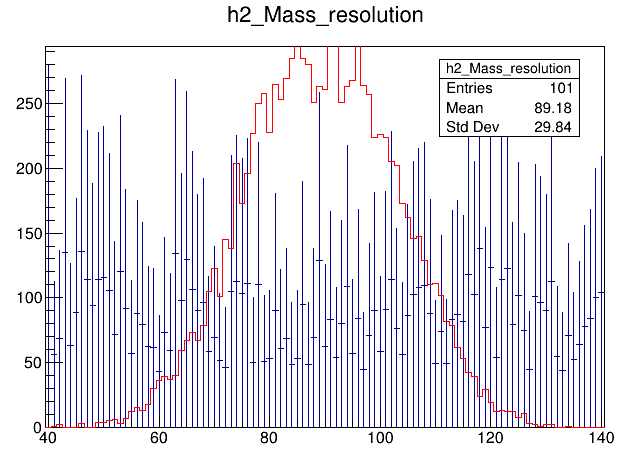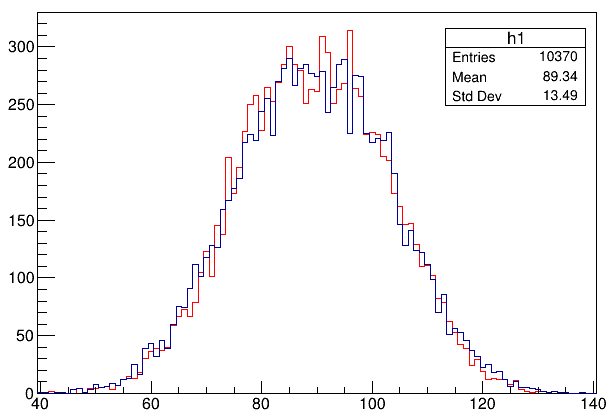# How to add experimental resolution to a 1D Histogram in root

Hi,
I am quite new to root.
Can someone tell me how to smear my theoritical mass spectra with my experimental mass resolution ?
The experimental mass resolution is 3.5 amu. I want to incorporate this mass resolution in the mass spectrum which I obtain from my theory calculation and want to see how the width changes.

Welcome to the ROOT forum.
Have you looked at the ROOT tutorials ? the ROOT forum ?

Yes, i have gone through the root tutorials and I had made a macro to do this. But It has some problems. i am sharing the marco, unfortunately I cant upload the root files.

``````void MassResolution()
{

TH1D*h2_Mass_resolution = new TH1D("h2_Mass_resolution","h2_Mass_resolution",101.,39.5,140.5); // Defined a histogram

TFile *file=TFile::Open("QnmFF_ROOT_Au_158.root");
TTree *tree=(TTree*)file->Get("DITY");

TH1D*h1 = new TH1D("h1","Os_187.46_MeV",101.,39.5,140.5);
h1->SetLineColor(kRed);
tree->Draw("Aff1>>h1"); // retrived histogram fom the TTree

Double_t x1,y1,z;
Double_t content=0;
Double_t sigma=3.5;

const UInt_t nX = h1->GetNbinsX();

for(UInt_t i=1;i<=nX;++i)
{
x1 = h1->GetXaxis()->GetBinCenter(i);
y1 = h1->GetBinContent(i);
z = h1->GetBinWidth(i);

TF1 *f1 = new TF1("f1","TMath::Gaus(x,,)",40,140);
f1->SetParameter(0,y1);
f1->SetParameter(1,sigma);

h2_Mass_resolution->Fill(x1, f1->GetRandom());

}
``````

which problems ?

that would help though …

Assuming that “`Aff1`” is in “amu” units and you want to add a Gaussian smearing with “3.5 amu” sigma, you could try:

``````tree->Draw("Aff1 + 3.5 * TMath::Sqrt2() * TMath::ErfInverse(2. * rndm() - 1.) >> h1"); // inverse Gaussian CDF transform sampling
``````The problem as you can see from the image, I am not getting the smeared histogram.
Red is my Theoritical data, to which I want to add my mass resolution of 3.5 amu. and when I run my macro I get the blue histogram which is not correct.

Since I am a new user of the root forum I am not able to upload my root file.

The method I proposed makes the “`h1`” histogram smeared (no need for any “`h2`” gymnastics).

I was just going through what you proposed. It is working, but I need to understand how you did it.Red is the theoretical data and blue is the theoretical data smeared to 3.5 amu.

Hi,
Inside my ttree I have all the theoretical masses for which I wanted to add my masss resolution of 3.5 amu. For that I declared a tree branch and variable “Aff1_Res_amu” and did

Aff1_Res_amu = Aff1 + 3.5 * TMath::Sqrt2() * TMath::ErfInverse(2. * rndm() - 1.);

When I do this I get the following error :

In a C++ code, instead of “`rndm()`” try to use “`gRandom->Rndm(1)`”.

Thank you for the prompt response !

So I replaced rndm(), like you toldAff1_Res_amu = Aff1 + 3.5 * TMath::Sqrt2() * TMath::ErfInverse(2. * gRandom->Rndm(1) - 1.);

but I get this error

I have used the header file " #include<TRandom.h>"

It seems you have an extra “g” in line 221 (column 1).

That was right, there was g. But there is still some problem with gRandom.

`if (!gRandom) gRandom = new TRandom3();`
`Aff1_Res_amu = gRandom->Gaus(Aff1, 3.5);`